# In a random sample of 32 large cap mutual funds, the average YTD return is 3.05%...

1. In a random sample of 32 large cap mutual funds, the average YTD return is 3.05% with a standard deviation of 9.77%. Conduct by hand an appropriate hypothesis test at the 5% level to test whether the average YTD return for a large cap mutual fund is positive. (Again, that means to write the hypotheses, compute the test statistic, and estimate the p-value.) Give your test conclusion in terms of the question being asked. Confirm your test statistic and result with Minitab. Also, interpret the associated confidence bound for this test.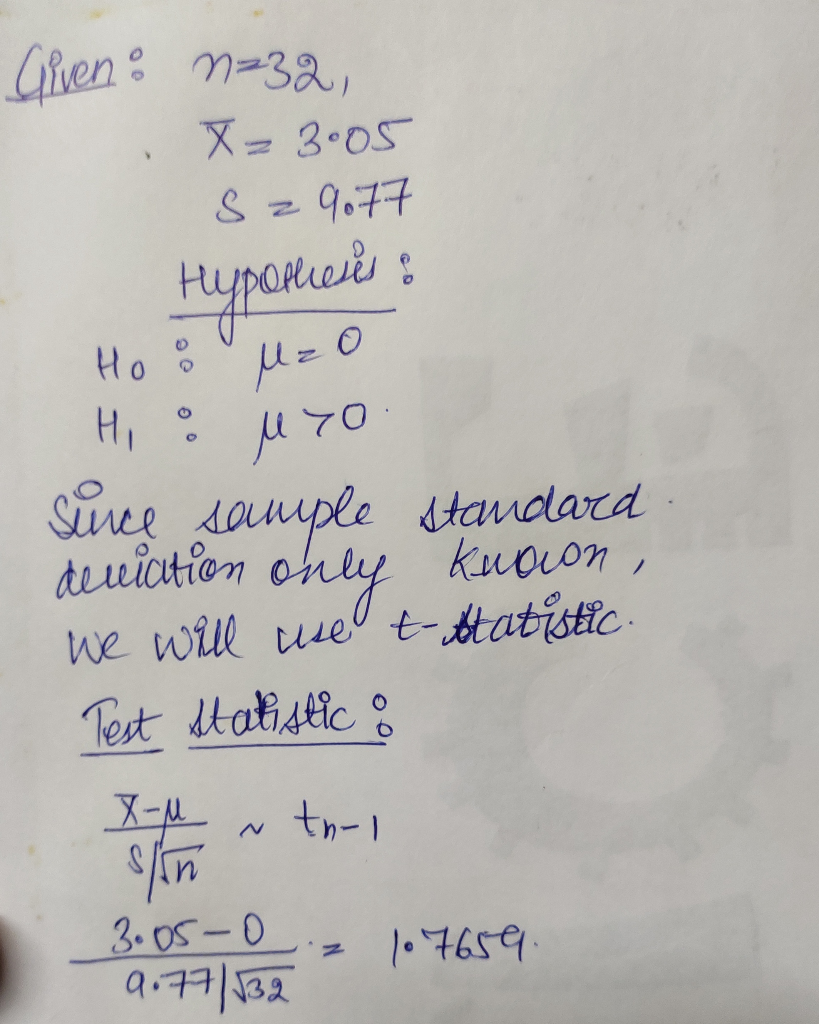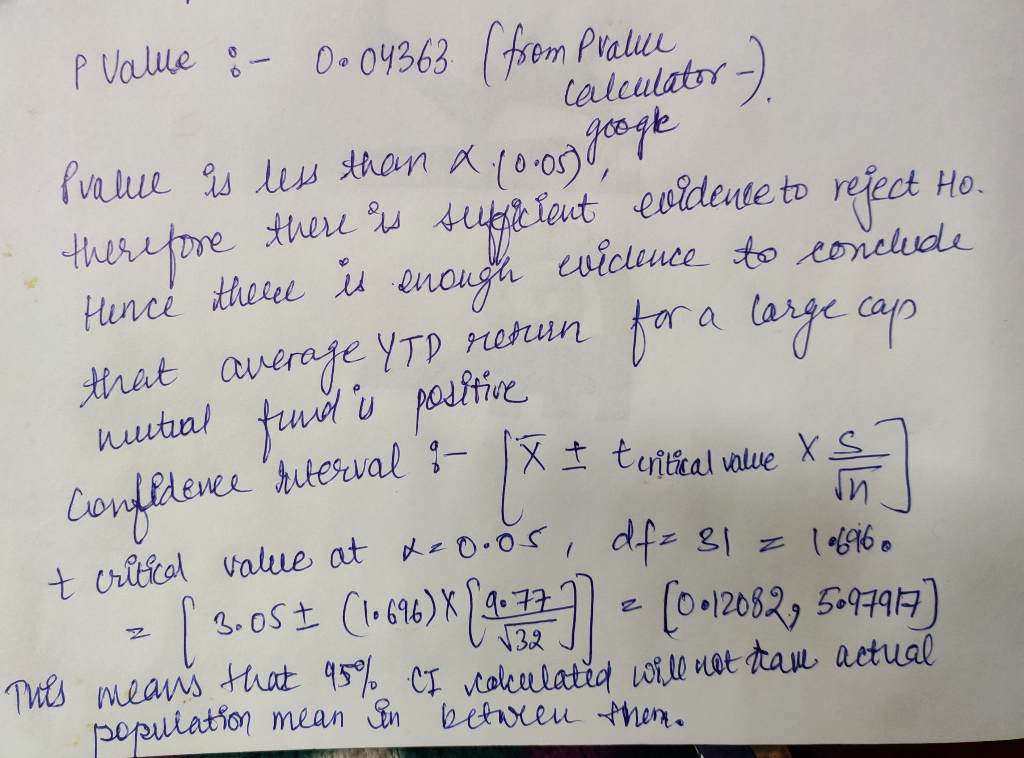From Minitab it can be done in the following steps:

Choose Stat > Basic Statistics > 1-Sample t

##### Add Answer to: In a random sample of 32 large cap mutual funds, the average YTD return is 3.05%...
Similar Homework Help Questions
• ### A sample of 55 mutual funds was taken and the mean return in the sample was...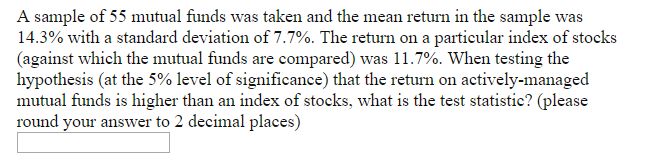A sample of 55 mutual funds was taken and the mean return in the sample was 14.3% with a standard deviation of 7.7%. The return on a particular index of stocks (against which the mutual funds are compared) was 11.7%. When testing the hypothesis (at the 5% level of significance) that the return on actively-managed mutual funds is higher than an index of stocks, what is the test statistic? (please round your answer to 2 decimal places)

• ### We are studying mutual bond funds for the purpose of investing in several funds

We are studying mutual bond funds for the purpose of investing in several funds. For this particular study, we want to focus on the assets of a fund and its five-year performance. The question is: Can the five-year rate of return be estimated based on the assets of the fund? Nine mutual funds were selected at random, and their assets and rates of return are shown below. a. Draw a scatter diagram. b. Compute the coefficient of correlation. c. Compute...

• ### Please help! Only Multiple Choice. 3. The average return from equity mutual funds over a period...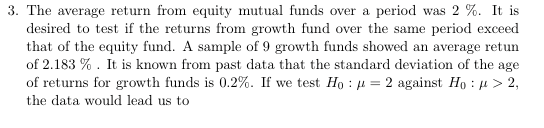Please help! Only Multiple Choice. 3. The average return from equity mutual funds over a period was 2 %. It is desired to test if the returns from growth fund over the same period exceed that of the equity fund. A sample of 9 growth funds showed an average retun of 2.183 % . It is known from past data that the standard deviation of the age of returns for growth funds is 0.2%. If we test H0 : μ-2...

• ### Using the dataset that you used for the midterm (Find it on the Blackboard), do the following: 1) (4 points) Submit the values for items a to e in the table below: mean, sample size, standard deviatio...

Using the dataset that you used for the midterm (Find it on the Blackboard), do the following: 1) (4 points) Submit the values for items a to e in the table below: mean, sample size, standard deviation, and 95% confidence interval for mean. (SPSS Command: Analyze/Descriptive Statistics/Explore) Variable Statistic Height Mean 71.2 Sample Size N 999 Standard Deviation 2.913 95% Confidence Interval for Mean Lower Bound 71.02 Upper Bound 71.38 2) (4 points) Assume that you want to test whether...

• ### A random sample of 22 STAT 250 students was collected and the file size of Data...

A random sample of 22 STAT 250 students was collected and the file size of Data Analysis 2 was recorded. The data was measured in megabytes. The instructors of the course claim that the file size will be different from 5 megabytes. Consider the population of all file sizes to be right-skewed. Using a = 0.01, is there sufficient evidence to conclude that the mean file size of Data Analysis 2 is different from 5 megabytes? Conduct a full hypothesis...

• ### Question 21 3 pts Large Sample Proportion Problem. Surveys were conducted in multiple countries and respondents...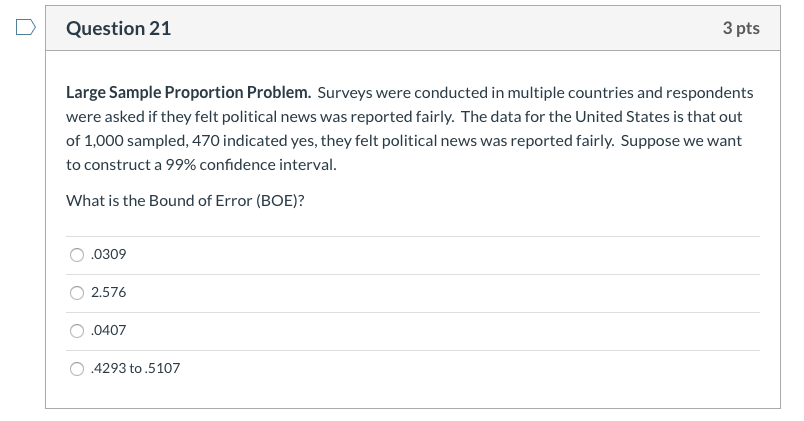Question 21 3 pts Large Sample Proportion Problem. Surveys were conducted in multiple countries and respondents were asked if they felt political news was reported fairly. The data for the United States is that out of 1,000 sampled, 470 indicated yes, they felt political news was reported fairly. Suppose we want to construct a 99% confidence interval. What is the Bound of Error (BOE)? 0.0309 2.576 0.0407 0.4293 to 5107 Question 22 3 pts Large Sample Proportion Problem. Surveys were...

• ### Among a simple random sample of 331 American adults who do not have a four-year college...

Among a simple random sample of 331 American adults who do not have a four-year college degree and are not currently enrolled in school, 48% said they decided not to go to college because they could not afford school. (a) A newspaper article states that only a minority of the Americans who decide not to go to college do so because they cannot afford it and uses the point estimate from this survey as evidence. Conduct a hypothesis test to...

• ### A random sample of 49 measurements from a population with population standard deviation o 3 had...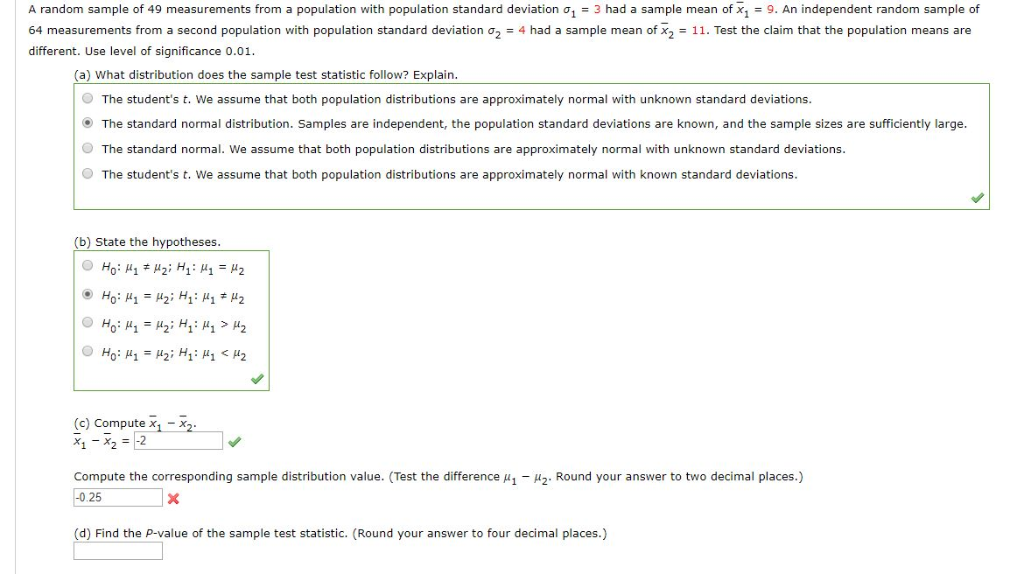A random sample of 49 measurements from a population with population standard deviation o 3 had a sample mean of x, 9. An independeent random sample of sample mean of x, 11. Test the claim that the population means are 64 measurements from a second population with population standard deviation a2 4 had different. Use level of significance 0.01. (a) What distribution does the sample test statistic follow? Explain. The student's t. We assume that both population distributions are approximately...

• ### Use the following information to complete steps (a) through (d) below. A random sample of ny...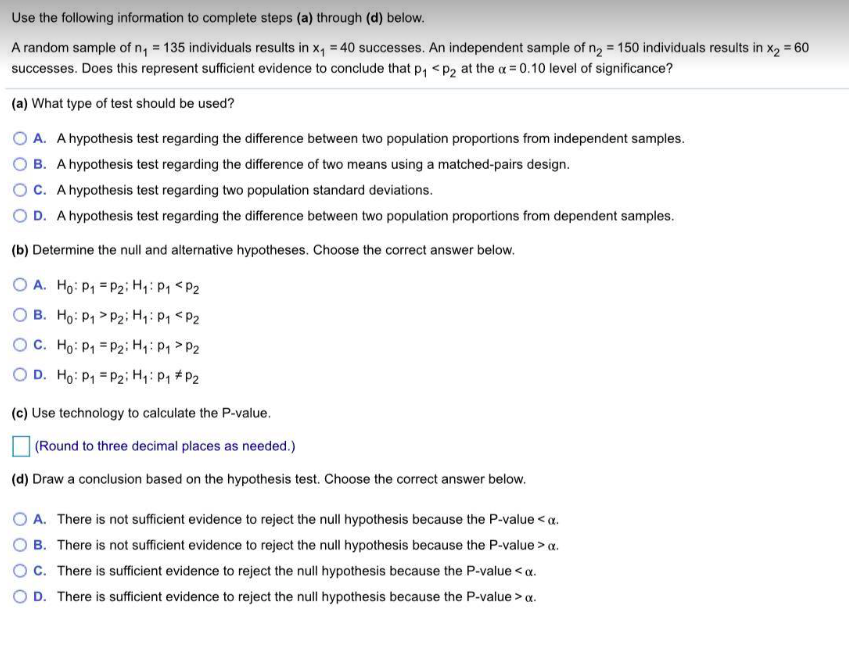Use the following information to complete steps (a) through (d) below. A random sample of ny = 135 individuals results in xy = 40 successes. An independent sample of n2 = 150 individuals results in x2 = 60 successes. Does this represent sufficient evidence to conclude that P, <P2 at the a = 0.10 level of significance? (a) What type of test should be used? A. A hypothesis test regarding the difference between two population proportions from independent samples. B....

• ### 1. A random sample of 30 boxes of bolts was purchased at a local hardware store...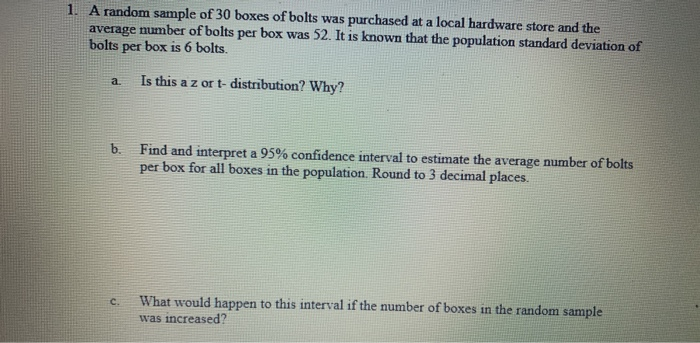1. A random sample of 30 boxes of bolts was purchased at a local hardware store and the average number of bolts per box was 52. It is known that the population standard deviation of bolts per box is 6 bolts. a Is this a z or t-distribution? Why? b. Find and interpret a 95% confidence interval to estimate the average number of bolts per box for all boxes in the population. Round to 3 decimal places. C What would...

Free Homework App